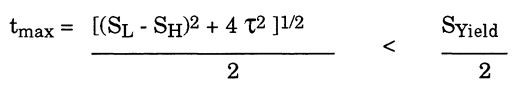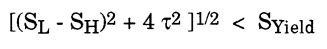Search

# 1.1.4 Maximum Stress Intensity Criterion

Most of the current piping codes use a slight modification of the maximum shear stress theory for flexibility related failures. Repeating, the maximum shear stress theory predicts that failure occurs when the maximum shear stress in a body equals Syjeld/2, the maximum shear stress existing at failure during the uniaxial tensile test. Recapping, the maximum shear stress in a body is given by:For the differential element at the outer surface of the pipe, the principal stresses were computed earlier as:As seen previously, the maximum shear stress theory states that during the uniaxial tensile test the maximum shear stress at failure is equal to one-half of the yield stress, so the following requirement is necessary:Multiplying both sides arbitrarily by two saves the time required to do two mathematical operations, without changing this relationship. Multiplying by two creates the stress intensity, which is an artificial parameter defined simply as twice the maximum shear stress. Therefore the Maximum Stress Intensity criterion, as adopted by most piping codes, dictates the following requirement:Note that when calculating only the varying stresses for fatigue evaluation purposes (as discussed in the following section), the pressure components drop out of the equation. If an allowable stress based upon a suitable factor of safety is used, the Maximum Stress Intensity criterion yields an expression very similar to that specified by the B31.3 code:Example Stress Intensity Calculations:

Calculation of stress intensity may be illustrated by returning to our 6-inch nominal diameter, standard wall pipe for which longitudinal, shear, and hoop stresses were calculated. Reviewing the results of those calculations:Assuming that the yield stress of the pipe material is 30,000 psi at temperature, and a factor of safety of 2/3 is to be used, the following calculations must be made:The 14674 psi is the calculated stress intensity in the pipe wall, while the 20000 is the allowable stress intensity for the material at the specified temperature. In this case, the pipe would appear to be safely loaded under these conditions.